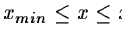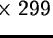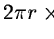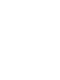Next: Backgrounds Up: 2-Dimensional Images Previous: 2-Dimensional Images

Patterns

Images BARS and OFFBARS, below, are of SCALE = 3; BARS30 and BARS60 have SCALE = 4; and RINGS and OFFRINGS have SCALE = 2. All of these images have CUTS defined as 0, 100. Reference may be made to Figs. 16.1 and 16.2 for a reproduction of these images, produced using the SET/SPLIT feature of MIDAS.

BARS.BDF

consists, firstly, of three rectangular bars; each of length 25 pixels; of width 1, 4, and 9 pixels; horizontally positioned; and flush with pixel boundaries. The uniform flux density in each pattern is 100 units per pixel. The three rectangular patterns are also vertically positioned, again flush with pixel boundaries. Note that because of this alignment of images and pixel grids and because of the dimensions of the images being in integer numbers of pixels -- which is not likely to occur in reality -- no effect of the spatial discretisation (i.e. of the necessarily finite pixel size) is visible.

In order to illustrate the case of a point spread function which in one dimension is extremely undersampled, a delta function is also included (the lower left hand pattern in BARS). It is defined as: f(x,y) = 100 where y = y0 and, for given y0, xmin and xmax. Note that the one-pixel wide pattern, flush with pixel boundaries, cannot be distinguished from the delta function.

OFFBARS.BDF

has the same patterns as BARS, but offset to a small amount in x and y relative to pixel boundaries. In the horizontal (x) direction, the offset is 0.4 of a pixel side length, and in the vertical (y) direction it is 0.2 of a pixel (both in the positive direction).

This yields for the rectangular patterns flux values of 32.0, 40.0, and 8.0 in the top left corner, along the boundary, and in the top right corner respectively; corresponding figures for the lower part of each pattern are: 48.0, 60.0, and 12.0; finally the flux along the left and right boundaries are 80.0 and 20.0. The comparison with BARS shows the effect of discrete steps quite clearly.

The delta function in OFFBARS is defined on a different interval of the x-axis. Its constant flux is apportioned to the pixels it runs through in the horizontal direction, but in the y (vertical axis) direction it has infinitesimal extension. In other words, shifting a delta function perpendicularly to the axis to which it is parallel has no effect while this is not true of the 1-pixel wide bar.

Note that flux conservation is at all times verified, both locally (single pixel) and globally.

BARS30.BDF

rotates the horizontal patterns of BARS through -30 degrees.

In all cases the center of rotation is the pixel in the lower left hand corner of the rectangular patterns. These pixel coordinates are 10, 20 and 34 pixel units distant from the delta function whose left hand pixel has coordinates (42,52).

The delta function is again taken as a line of given starting point, of given length, of given angle to the horizontal, and of infinitesimal width. Note the difference between this and the 1-pixel wide bar.

In order not to depend on any interpolation algorithms etc., creation of these patterns was carried out by subdividing pixels, rotating the subpixels, and accumulating flux contributions of these subpixels. For a subdivision ofthe elapsed time may be a clearer indicator of reconstruction time: BARS30 took approximately 30 hours on a VAX 11/785 machine.

An estimate of precision was obtained in the following way. For the central portion of the larger rectangles, flux of 100 ought to obtained. If pixel subdivision is coarse, values greater than or less than 100 will be obtained. Hence, the sampling of pixels where the value 100 is expected allows an estimate of accuracy, and should additionally vary proportionally to n2 where pixel subdivision is. (The latter statement is based on empirically noting that a small constant number of subpixels -- usually 1 -- were scattered'' in being projected. This n2 precision dependence was empirically verified.) For n = 299, accuracy defined in this way was determined as 0.003 units, i.e. in no pixel the residual from 100 units is larger than this value.

BARS60.BDF

was constructed in an analogous manner, with the same precision, as BARS30. In this case, the angle of rotation was +60 degrees. The coordinates of the left hand pixel of the delta function are again (42,52), and the other patterns have lower left hand pixels distant by 10, 20 and 34 pixel units.

RINGS.BDF

relates to centered, circular patterns. The patterns here are a delta function (infinitely thin) at radius 10 units, and rings of thicknesses 1, 5 and 10 units at inner surface radii 22, 33 and 48 steps. Uniform flux density of 100 units/pixel is used. Additionally flux 100 is located in the pixel at the center of the set of concentric rings. Note again the difference between the delta function and the ring of thickness 1: the former distributes flux (100 units) to any pixel which is overlapped by a mathematically-defined circular curve; while the ring of thickness 1 distributes flux to pixels proportionally to their overlap with the ring.

Total flux in the delta function was defined as, and subdivision was carried out in intervals of 0.025 degree.

Technically, the approach adopted in constructing these patterns was to subdivide pixels, rotate (or otherwise project) the small subpixels, and accumulate the flux (cf. BARS30 and BARS60). This allows the precision of the annuli to be assessed by noting that the approximation improves as the subpixels get smaller, and that the typical flux excess or deficit in one output pixel amounts to the flux content of one subpixel. Per output pixel, this gives a maximum error of(where n2 subpixels correspond to the original pixel with flux 100), and a mean error of half this value (where we assume uniform statistical fluctuation; a histogram analysis of the frames created confirms this). For n = 101, we therefore obtain a maximum error estimate of less than 0.01 units.

RINGS is perfectly symmetric under rotation through multiples of 90 degrees.

OFFRINGS.BDF

is analogous to RINGS, except offset from the center of a pixel by (0.3,0.25) units. Note the effect of this offset on the flux distributed uniformly in a square of pixel dimensions at the center; and note also that shifting the annuli as done in OFFRINGS necessarily destroys the symmetry of the annuli. In other respects (time required for construction, precision) OFFRINGS presents similar characteristics to RINGS.Next: Backgrounds Up: 2-Dimensional Images Previous: 2-Dimensional Images
Petra Nass
1999-06-15